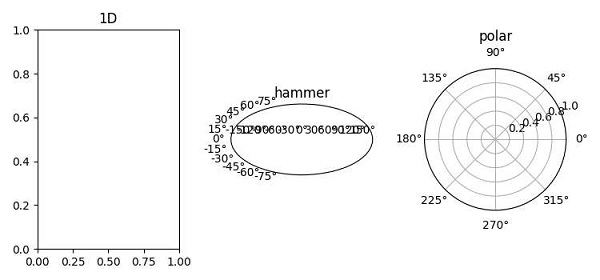# How do I change matplotlib's subplot projection of an existing axis?

It seems difficult to change the projection of an existing axis, but we can take the following steps to create different type projections −

• Using subplot() method, add a subplot to the current figure, with nrows=1, ncols=3 and current index=1.

• Add a title to the current axis.

• Using subplot() method, add a subplot to the current figure, with nrows=1, ncols=3 and current index=2, projection=hammer.

• Add a title to current axis, hammer.

• Using subplot() method, add a subplot to the current figure, with nrows=1, ncols=3 and current index=3, projection=polar.

• Add a title to current axis, polar.

• To display the figure, use the show() method.

## Example

from matplotlib import pyplot as plt
plt.rcParams["figure.figsize"] = [7.50, 3.50]
plt.rcParams["figure.autolayout"] = True
plt.subplot(131)
plt.title("1D")
plt.subplot(132, projection="hammer")
plt.title("hammer")
plt.subplot(133, projection="polar")
plt.title("polar")
plt.show()

## Output